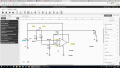# High end current sensing device , 12V dc to detect load on or not

#### Fabio Yau

Joined Jul 29, 2018
1
Designed the below circuit to have a 0.1 ohm shunt to detect the load on with 250mA current.
And load off without shunt current ( shunt voltage ) to drive the V out at low ( 0) voltage
Shunt voltage = 250mA x 0.1 = 25mV , with 1000K ohm to 10K ohm = 100 times
The voltage output should be 100 x 25mv = 2.5 Voltage

1) the V out resulted equal to zero ? Why ? Not 2.5V with 100 times 25mv
2) the inverting input should be 12V /2 = 6V , but it was 9.87V. Why?
3) Ideally, the non inverting input should be the same as inverting that was 9.87V. But it was 6.99V. Why ?#### bertus

Joined Apr 5, 2008
21,940
Hello,

For a difference measurement R4 and R6 should be equal and also R3 and R5 sould be equal.

Bertus

#### crutschow

Joined Mar 14, 2008
30,116
Two main problems:

For a differential amp to work properly R4 and R6 must have the same value (look up differential amp).
So if you want a differential gain of 100, both R4 and R6 must be 1000kΩ (1megΩ).

Also the input common-mode range of the LM2904 is not high enough to work in that circuit (the common-mode voltage will be 12V(1000k/1010k) = 11.88V).
You need a rail-rail type op amp.